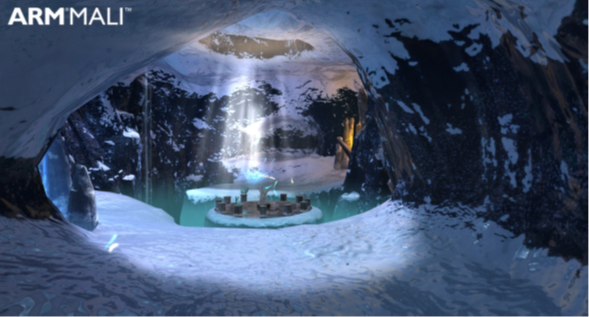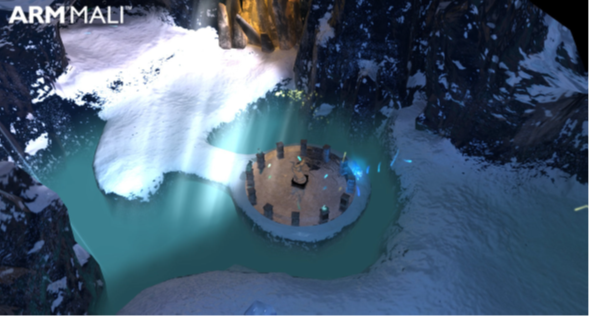## Dynamic soft shadows based on local cubemaps

Dynamic soft shadows based on local cubemaps uses a local cubemap to hold a texture that represents transparency of the static environment. This technique a very efficient technique that generates high-quality soft shadows.

In your scene, there are moving objects and static environments like rooms. By using this technique, you are not required to render static geometry to a shadow map every frame. This technique enables you to use a texture to represent the shadows.

Cubemaps can be a good approximation of a static local environment, including irregular shapes like the cave in the Ice Cave demo. The alpha channel can also represent the amount of light that enters the room.

The objects that move are typically everything except the room, for example:

• The sun
• The camera
• Dynamic objects

When the whole room is represented by a cube texture, you can access arbitrary texels of the environment within a fragment shader. For example, the sun can be in any arbitrary position and you can calculate the amount of light reaching a fragment based on the value fetched from the cubemap.

The alpha channel, or transparency, represents the amount of light that enters the local environment. In your scene, pass the cubemap texture through to the fragment shaders that render the static and dynamic objects that want shadows added.

#### Generate shadow cubemaps

The following instructions describe how to generate shadow cubemaps:

1. Start with a local environment that you want to add shadows to, from light sources outside of the environment. For example, a room, a cave, or a cage.
2. This technique is like reflections based on a local cubemap. For more information, see Implement reflections with a local cubemap.
3. Create the shadow cubemap in the same way that you created the reflection cubemap, then add an alpha channel. This channel represents the amount of light that enters the local environment.
4. Calculate the position to render the six faces of the cubemap from. Usually the render position is in the center of the bounding box of the local environment. This position is required for the generation of the cubemap. The position also must be passed to the shaders to calculate a local correction vector to fetch the right texel from the cubemap.
5. When you have decided where to position the center of the cubemap you can render all the faces to the cubemap texture. This means that you can record the transparency, or alpha, of the local environment. The more transparent an area is, the more light comes into the environment. If there is no geometry, the texture is fully transparent. If necessary, you can use the RGB channels to store the color of the environment for colored shadows, for example stained glass, reflections, or refractions.

#### Render shadows

The following instructions briefly describe how to render shadows:

1. Build a vector `PiL` in world space from a vertex or fragment, to the light or lights, and fetch the cubemap shadow using this vector.
2. Before fetching each texel, you must apply local correction to the `PiL` vector.
Note: Arm recommends you make the local correction in the fragment shader to obtain more accurate shadows.
3. To compute the local correction, you must calculate an intersection point of the fragment-to-light vector with the bounding box of the environment. Use this intersection point to build another vector from the cubemap origin position `C` to the intersection point `P`. This computes the output value `CP`, which is the corrected fragment-to-light vector that you use to fetch a texel from the shadow cubemap.

The following input parameters are required to calculate the local correction:

`_EnviCubeMapPos`
The cubemap origin position
`_BBoxMax`
The maximum point of the bounding box of the environment
`_BBoxMin`
The minimum point of the bounding box of the environment
`Pi`
The fragment position in world space
`PiL`
The normalized fragment-to-light vector in world space

The following image shows the local correction of the fragment-to-light vector:The following example code shows how to calculate the correct `CP` vector:

```// Working in World Coordinate System.
vec3 intersectMaxPointPlanes = (_BBoxMax - Pi) / PiL;
vec3 intersectMinPointPlanes = (_BBoxMin - Pi) / PiL;
// Looking only for intersections in the forward direction of the ray.
vec3 largestRayParams = max(intersectMaxPointPlanes, intersectMinPointPlanes);
// Smallest value of the ray parameters gives us the intersection.
float dist = min(min(largestRayParams.x, largestRayParams.y), largestRayParams.z);
// Find the position of the intersection point.
vec3 intersectPositionWS = Pi + PiL * dist;
// Get the local corrected vector.
CP = intersectPositionWS - _EnviCubeMapPos;
```

Use the `CP` vector to fetch a texel from the cubemap. The alpha channel of the texel provides information about how much light or shadow you must apply to a fragment:

`float shadow = texCUBE(cubemap, CP).a;`

The following image shows a chess room with hard shadows: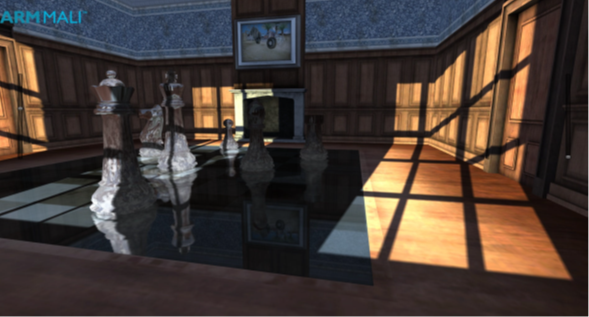This technique generates working shadows in your scene, but you can improve the quality of the shadows with two more steps:

• Back faces in shadow
• Smoothness
Back faces in shadow
The cubemap shadow technique does not use depth information to apply shadows. Therefore, some faces are incorrectly lit when they are meant to be in shadow.
The problem only occurs when a surface is facing in the opposite direction to the light. To fix this problem, check the angle between the normal vector and the fragment-to-light vector, `PiL`. If the angle, in degrees, is outside of the range -90 to 90, the surface is in shadow.
The following code snippet checks to see if it outside the range:
`if (dot(PiL,N) < 0) shadow = 0.0;`
The preceding code causes a hard switch from light to shade. For a smoother transition, use the following formula:
`shadow *= max(dot(PiL, N), 0.0);`
Here is what the formula does:
• `shadow` is the alpha value fetched from the shadow cubemap
• `PiL` is the normalized fragment-to-light vector in world space
• `N` is the normal vector of the surface in world space
The following image shows a chess room with back faces in shadow: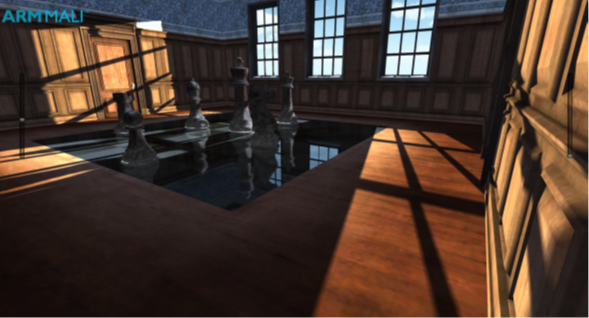Smoothness
This shadow technique can provide realistic soft shadows in your scene. The following steps explain how you can apply smoothness:
1. Generate mipmaps and set trilinear filtering for the cubemap texture.
2. Measure the length of a fragment-to-intersection-point vector.
3. Multiply the length by a coefficient.
The coefficient is a normalizer of a maximum distance in your environment to the number of mipmap levels. You can calculate the coefficient automatically against bounding volume and mipmap levels. You must customize the coefficient to your scene, enabling you to tweak the settings to suit the environment, improving visual quality. For example, the coefficient used in the Ice Cave project is 0.08.
You can reuse the results from the calculations that you did for the local correction. This is shown in the following steps:
1. Create a variable `texLod` for the length of the segment from the fragment position to the intersection point of the fragment-to-light vector with the bounding box. Set this variable to `dist` from the code snippet for local correction.
2. This is shown in the following code:
`float texLod = dist;`
3. Multiply `texLod` by the distance coefficient:
`texLod *= distanceCoefficient;`
4. To implement softness, fetch the correct mipmap level of the texture using the Cg function `texCUBElod()` or the GLSL function `textureLod()`.
5. Construct a `vec4` where `XYZ` represents a direction vector and the `W` component represents the LOD, as shown in the following code:
```CP.w = texLod;
shadow = texCUBElod(cubemap, CP).a;```
6. This technique provides high-quality, smooth shadows for your scene.

The following image shows a chess room with smooth shadows: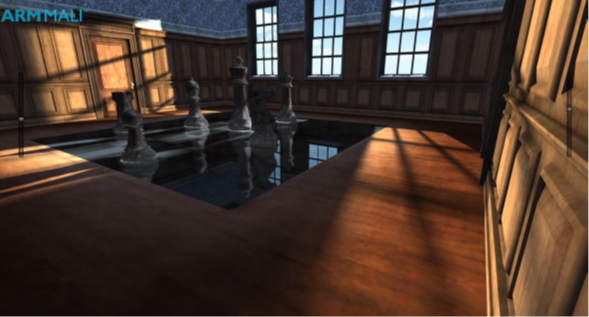#### Combine cubemap shadows with a shadow map

This section describes combining cubemap shadows with a shadow map.

To get shadows that are complete with dynamic content, you must combine cubemap shadows with a traditional shadow map technique. Combing cubemap shadows with a traditional shadow map technique is more work, but it is worth the extra work because you are only required to render dynamic objects to the shadow map. This is shown when comparing the following two images:

The following image shows the chess room with smooth shadows only: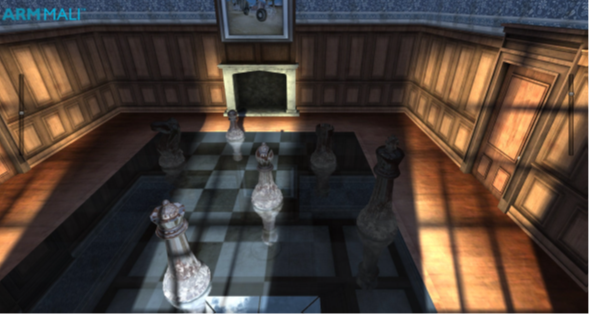The following image shows the chess room with smooth shadows combined with dynamic shadows: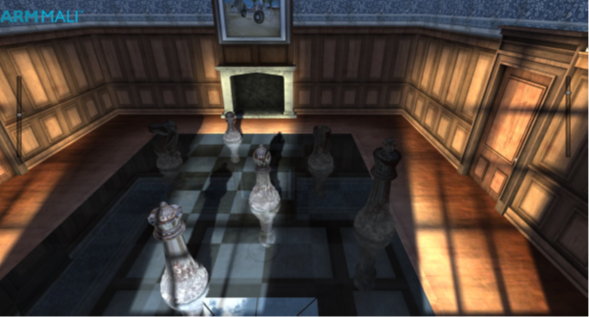#### Results of the cubemap shadow technique

This section shows you the results of the cubemap shadow technique, which was introduced to you in Combine cubemap shadows with a shadow map.

In traditional techniques, rendering shadows can be quite expensive because it involves rendering the whole scene from the perspective of each shadow-casting light source. The cubemap shadow technique described here delivers improved performance because it is mostly pre-baked.

The cubemap shadow technique is also independent of output-resolution. It produces the same visual quality at 1080p, 720p, and other resolutions.

The softness filtration is calculated in hardware, so the smoothness comes at almost no computational cost. The smoother the shadow, the more efficient the technique is. This is because the smaller mipmap levels result in less data than traditional shadow map techniques. Traditional techniques require a large kernel to make shadows smooth enough to be more visually appealing. Therefore, this requires high memory bandwidth, therefore, reducing performance.

The quality that you get with the cubemap shadow technique is higher than you might expect. It provides realistic softness and stable shadows with no shimmering at the edges. Shimmering edges can be observed when using traditional shadow map techniques because of rasterization and aliasing effects. However, none of the anti-aliasing algorithms can fix this problem entirely.

The cubemap shadow technique does not have a shimmering problem. The edges are stable even if you are using a much lower resolution than the one that is used in the render target. You can use four times lower resolution than the output and there are not artifacts or unwanted shimmering. Using four times lower resolution also saves memory bandwidth, therefore, improving performance.

Note: This technique can be used on any device on the market that supports shaders like OpenGL ES 2.0 or higher. It combines well with the reflections that are based on local cubemaps technique, making shadows an easy addition.

Note: The technique cannot be used for everything in your scene. Dynamic objects, for instance, receive shadows from the cubemap but they cannot be pre-baked to the cubemap texture. For dynamic objects, use shadow maps for generating shadows, blended with the cubemap shadow technique.

The following image shows the Ice Cave with shadows: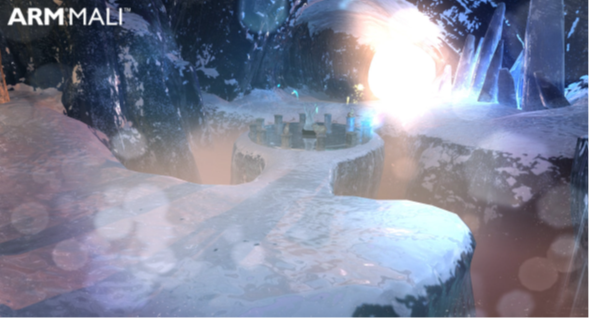The following images show the Ice Cave with smooth shadows: